[This article was first published on R - Data Science Heroes Blog, and kindly contributed to R-bloggers]. (You can report issue about the content on this page here)
Want to share your content on R-bloggers? click here if you have a blog, or here if you don't.Hi there 🙂

This new package –install.packages("funModeling")– tries to cover with simple concepts common tasks in data science. Written like a short tutorial, its focus is on data interpretation and analysis.

Below, you’ll find a copy-paste from the package vignette, (so you can drink a good coffee while you read it… )

#### Introduction

This package covers common aspects in predictive modeling:

1. Data Cleaning
2. Variable importance analysis
3. Assessing model performance

Main purpose of this package is to teach some predictive modeling using a practical toolbox of functions and concepts, to people who is starting in data science, small data and big data. With special focus on results and analysis understanding.

#### Part 1: Data cleaning

Overview: Quantity of zeros, NA, unique values; as well as the data type may lead to a good or bad model. Here an approach to cover the very first step in data modeling.

## Loading needed libraries
library(funModeling)
data(heart_disease)

###### Checking NA, zeros, data type and unique values
my_data_status=df_status(heart_disease)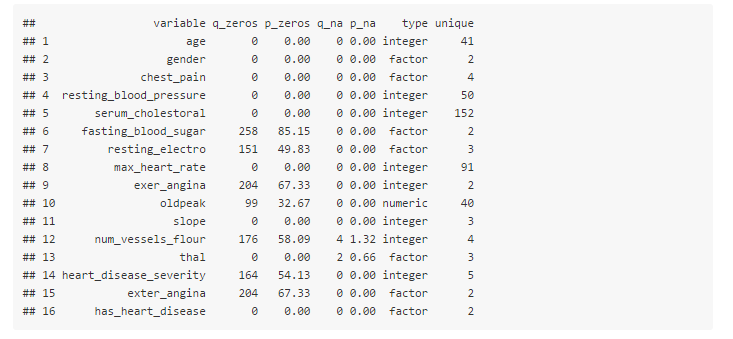• q_zeros: quantity of zeros (p_zeros: in percentage)
• q_na: quantity of NA (p_na: in percentage)
• type: factor or numeric
• unique: quantity of unique values
###### Why are these metrics important?
• Zeros: Variables with lots of zeros may be not useful for modeling, and in some cases it may dramatically bias the model.
• NA: Several models automatically exclude rows with NA (random forest, for example). As a result, the final model can be biased due to several missing rows because of only one variable. For example, if the data contains only one out of 100 variables with 90% of NAs, the model will be training with only 10% of original rows.
• Type: Some variables are encoded as numbers, but they are codes or categories, and the models don’t handle them in the same way.
• Unique: Factor/categorical variables with a high number of different values (~30), tend to do overfitting if categories have low representative, (decision tree, for example).
###### Filtering unwanted cases

Function df_status takes a data frame and returns a the status table to quickly remove unwanted cases.

Removing variables with high number of NA/zeros

# Removing variables with 60% of zero values
vars_to_remove=subset(my_data_status, my_data_status$p_zeros > 60) vars_to_remove["variable"]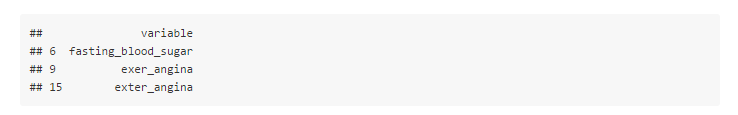## Keeping all except vars_to_remove heart_disease_2=heart_disease[, !(names(heart_disease) %in% vars_to_remove[,"variable"])]  Ordering data by percentage of zeros my_data_status[order(-my_data_status$p_zeros),]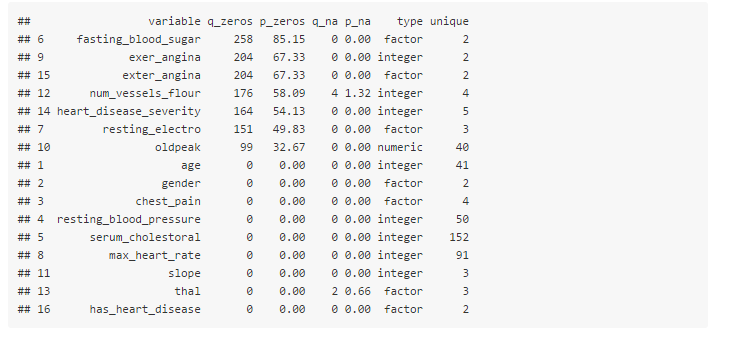#### Part 2: Variable importance with cross_plot

• Overview:
• Analysis purpose: To identify if the input variable is a good/bad predictor through visual analysis.
• General purpose: To explain the decision of including -or not- a variable to a model to a non-analyst person.

Constraint: Target variable must have only 2 values. If it has NA values, they will be removed.

Note: Please note there are many ways for selecting best variables to build a model, here is presented one more based on visual analysis.

###### Example 1: Is gender correlated with heart disease?
cross_gender=cross_plot(heart_disease, str_input="gender", str_target="has_heart_disease")Last two plots have the same data source, showing the distribution of has_heart_disease in terms of gender. The one on the left shows in percentage value, while the one on the right shows in absolute value.

###### How to extract conclusions from the plots? (Short version)

Gender variable seems to be a good predictor, since the likelihood of having heart disease is different given the female/male groups. it gives an order to the data.

###### How to extract conclusions from the plots? (Long version)

From 1st plot (%):

1. The likelihood of having heart disease for males is 55.3%, while for females is: 25.8%.
2. The heart disease rate for males doubles the rate for females (55.3 vs 25.8, respectively).

From 2nd plot (count):

1. There are a total of 97 females:

• 25 of them have heart disease (25/97=25.8%, which is the ratio of 1st plot).
• the remaining 72 have not heart disease (74.2%)
2. There are a total of 206 males:

• 114 of them have heart disease (55.3%)
• the remaining 92 have not heart disease (44.7%)
3. Total cases: Summing the values of four bars: 25+72+114+92=303.

Note: What would it happened if instead of having the rates of 25.8% vs. 55.3% (female vs male), they had been more similar like 30.2% vs. 30.6%). In this case variable gender it would have been much less relevant, since it doesn’t separate the has_heart_disease event.

###### Example 2: Crossing with numerical variables

Numerical variables should be binned in order to plot them with an histogram, otherwise the plot is not showing information, as it can be seen here:

###### Equal frequency binning

There is a function included in the package (inherited from Hmisc package) : equal_freq, which returns the bins/buckets based on the equal frequency criteria. Which is -or tries to- have the same quantity of rows per bin.

For numerical variables, cross_plot has by default the auto_binning=T, which automtically calls the equal_freq function with n_bins=10 (or the closest number).

cross_plot(heart_disease, str_input="max_heart_rate", str_target="has_heart_disease")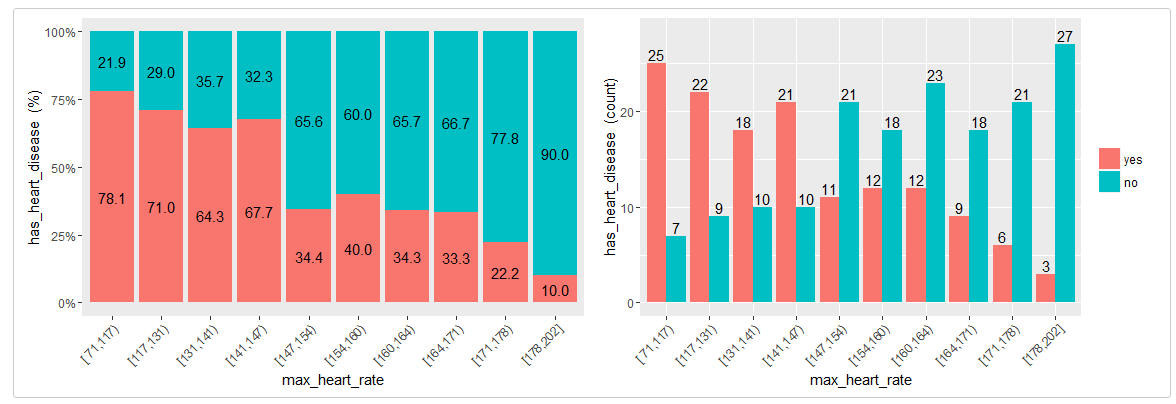###### Example 3: Manual binning

If you don’t want the automatic binning, then set the auto_binning=F in cross_plot function.

For example, creating oldpeak_2 based on equal frequency, with 3 buckets.

heart_disease$oldpeak_2=equal_freq(var=heart_disease$oldpeak, n_bins = 3)
summary(heart_disease$oldpeak_2)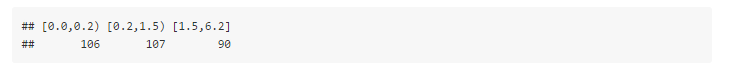Plotting the binned variable (auto_binning = F): cross_oldpeak_2=cross_plot(heart_disease, str_input="oldpeak_2", str_target="has_heart_disease", auto_binning = F)###### Conclusion This new plot based on oldpeak_2 shows clearly how: the likelihood of having heart disease increases as oldpeak_2 increases as well. Again, it gives an order to the data. ###### Example 3: Noise reducing Converting variable max_heart_rate into a one of 10 bins: heart_disease$max_heart_rate_2=equal_freq(var=heart_disease$max_heart_rate, n_bins = 10) cross_plot(heart_disease, str_input="max_heart_rate_2", str_target="has_heart_disease")At a first glance, max_heart_rate_2 shows a negative and linear relationship, however there are some buckets which add noise to the relationship. For example, the bucket (141, 146] has a higher heart disease rate than the previous bucket, and it was expected to have a lower. This could be noise in data. Key note: One way to reduce the noise (at the cost of losing some information), is to split with less bins: heart_disease$max_heart_rate_3=equal_freq(var=heart_disease\$max_heart_rate, n_bins = 5)
cross_plot(heart_disease, str_input="max_heart_rate_3", str_target="has_heart_disease")Conclusion: As it can be seen, now the relationship is much clean and clear. Bucket ‘N’ has a higher rate than ‘N+1’, which implies a negative correlation.

How about saving the cross_plot result into a folder? Just set the parameter path_out with the folder you want -It creates a new one if it doesn’t exists-.

cross_plot(heart_disease, str_input="max_heart_rate_3", str_target="has_heart_disease", path_out="my_plots")


It creates the folder my_plots into the working directory.

###### Example 4: cross_plot on multiple variables

Imagine you want to run cross_plot for several variables at the same time. To achieve this goal you define a list of strings containing all the variables to use as input in the cross_plot, and then, call the function massive_cross_plot.

If you want to analyze these 3 variables:

vars_to_analyze=c("age", "oldpeak", "max_heart_rate")

massive_cross_plot(data=heart_disease, str_target="has_heart_disease", str_vars=vars_to_analyze)


Automatically saving all the results into a folder Same as cross_plot, this function has the path_out parameter.

massive_cross_plot(data=heart_disease, str_target="has_heart_disease", str_vars=vars_to_analyze, path_out="my_plots")

###### Final notes:
• Correlation does not imply causation
• cross_plot is good to visualize linear relationships, giving it a hint on non-linear relationships.
• Cleaning the variables help the model to better modelize the data.

#### Part 3: Assessing model performance

Overview: Once the predictive model is developed with training data, it should be compared with test data (which wasn’t seen by the model before). Here is presented a wrapper for the ROC Curve and AUC (area under ROC) and the KS (Kolmogorov-Smirnov).

###### Creating the model
## Training and test data. Percentage of training cases default value=80%.
index_sample=get_sample(data=heart_disease, percentage_tr_rows=0.8)

## Generating the samples
data_tr=heart_disease[index_sample,]
data_ts=heart_disease[-index_sample,]

## Creating the model only with training data
fit_glm=glm(has_heart_disease ~ age + oldpeak, data=data_tr, family = binomial)

###### ROC, AUC and KS performance metrics
## Performance metrics for Training Data
model_performance(fit=fit_glm, data = data_tr, target_var = "has_heart_disease")## Performance metrics for Test Data
model_performance(fit=fit_glm, data = data_ts, target_var = "has_heart_disease")Key notes

• The higher the KS and AUC, the better the performance is.
• KS range: from 0 to 1.
• AUC range: from 0.5 to 1.
• Performance metrics should be similar between training and test set.

• KS and AUC focus on similar aspects: How well the model distinguishes the class to predict.
• ROC and AUC article: link